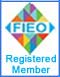Note: No part of this website may be reproduced, stored in a retrieval, or transmitted in any form or by any mean

## Our ProductsHome » Physics Laboratory Instruments   »   Boltzman Constant Kit Boltzman Constant Kit Objective: MEASUREMENT of Boltzmans Constant using semiconductor Diode.Boltzmann Constant Kit Code: BCK-001 Boltzman Constant KitConventional method to determine Boltzman Constant (k) make use of the block body Radiation and its two famous laws i.e. Wiens displacement law and Stefans law . These methods are time consuming and very often lead to comparatively less accurate results. The V  I characteristics of a semiconductor diode can be used to determine Boltzman Constant accurately and with simple equipment that can be handled with ease and convenience . Principle : The diode equation is given by:where V = voltage across the diode I = forward current at voltage V Io = reverse saturation current k = Boltzmann Constant T = Temperature in Kelvin n = a constant , characteristic of the material from which the diode is made , for Ge diode, n = 1 while n = 2 for Si diode. If V >> kT/e , the Boltzmann constant can be expressed aswhereis the slope of the straight line drawn between V and ln(I) INSTRUMENT The experimental kit provides the following facilities : (a) A digital millivoltmeter (0  9.99 V) to measure the voltage across the diode. (b) A highly stabilized power supply whose voltage can be varied in steps of 1 mV using a ten  turn potentiometer. (c) A digital current ammeter ( 0  500 mA). (d) Diodes: Silicon diodes & Germanium diodes.